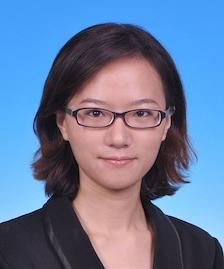# Assistant Professor Yan-Li Wang

## Mechanics

Office Location
Room B213
Telephone
86-10-56981984
Email
ylwang[at]csrc.ac.cn
Education

2014, PHD. Peking University, Beijing, China

2009, B.Sc. Jilin University, Changchun, China

Professional Employment

Yanli Wang received her PhD (2014) under supervision of Prof. Ruo Li. Before joining CSRC, she

worked as an assistant professor in Institute of Applied Physics and Computational Mathematics,

Beijing, China. In 2019, she joined CSRC (Beijing) as a Research Assistant Professor.

Research Interests

Model Reduction of the Kinetic Equations

Regularized Moment Method

Numerical Simulation of Multi-phase Fluid Flow

Awards/Honors

2013, Leo KoGuan Scholarship, Peking University

2009-2013, Scholarship at Peking University

2005-2008, First-class Academic Scholarship at Jilin University

Representative Publications
1.  Z.N. Cai, R. Li and Y.L. Wang. (2012) An Efficient NRxx Method for Boltzmann-BGK Equation. Journal of Scientific Computing, 50, pp. 103-119.
2.  Z.N. Cai, R. Li and Y.L. Wang. (2012) Numerical Regularized Moment Method for High Mach Number Flow. Communication in Computational Physics, 11 (5), pp. 1415-1438.
3.  Z. N. Cai, Y.W. Fan, R. Li, T. Lu and Y.L. Wang. (2012) Quantum Hydrodynamic Model by Moment Closure of Wigner Equation. Journal of Mathematical Physics, 53 (10), pp. 103503.
4.  R. Li, T. Lu, Y.L. Wang and W.Q. Yao. (2014) Numerical Validation for High Order Hyperbolic Moment System of Wigner Equation. Communications in Computational Physics, 15 (3), pp. 569-595.
5.  Z.C. Hu, R. Li, T. Lu, Y.L. Wang and W.Q. Yao. (2014) Simulation of an n+-n-n+ Diode by Using Globally-Hyperbolically-Closed High-Order Moment Models. Journal of Scientific Computing, 59 (3), pp. 761-774.
6.  Z.N. Cai, R. Li and Y.L. Wang. (2014) Solving Vlasov Equations Using NRxx Method. SIAM Journal on Scientific Computing, 35 (6), pp. A2807-A2831.
7.  Y.L. Wang and S.D. Zhang. (2017) Solving Vlasov-Poisson-Fokker-Planck Equations using NRxx Method. Communication in Computational Physics. 21 (3), pp. 782-807.
8.  Z.N. Cai, and Y.L. Wang. (2018) Suppressing Recurrence in Advection Equations. SIAM Journal on Numerical Analysis. 56 (5), pp. 3144-3168.
9.  Y.N. Di, R. Li, Z.Z Kou and Y.W. Fan and Y.L. Wang. (2019) Filtered Hyperbolic Moment Method in Vlasov Equations. Journal of Scientific Computing. 79 (2), pp. 969–991.
10.  Y.L. Wang and Z.N. Cai. (2019) Approximation of the Boltzmann collision operator based on Hermite spectral method. Journal of Computational Physics, 397, pp. 108815.
11.  R. Li, Y.L. Wang and C.B. Yao. (2020) A Robust Riemann Solver for Multiple Hydro-Elastoplastic Solid Mediums, Advances in Applied Mathematics and Mechanics, 12, pp. 212-250.
12.  Z.N. Cai, Z.C. Hu and Y.L. Wang. (2020) Numerical Simulation of Microflows Using Hermite Spectral Methods. SIAM Journal on Scientific Computing, 42 (1), pp. B105-B134.
13.  Z.N. Cai, Y.W. Fan and Y.L. Wang. (2020) Burnett Spectral Method for the Spatially Homogeneous Boltzmann Equation. Computers & Fluids, 300(20), pp. 104456.
14.  R. Li, Y.L. Wang and Y.X. Wang. (2020) Approximation to Singular Quadratic Collision Modelin Fokker-Planck-Landau Equation. (accepted by SIAM Journal on Scientific Computing)
15.   Z.N. Cai and Y.L. Wang. (2020) R13 Equations for Inverse Power Law Models. (accepted by Journal of Fluid Mechanics)
16.   R. Li, Y.N. Ren and Y.L. Wang. (2020) Hermite spectral method for Fokker-Planck-Landau equation modeling collisional plasma. (submitted)
17.   W.M. Li, P. Song and Y.L. Wang. (2020) A hybrid moment method for multi-scale kinetic equations based on maximum entropy principle. (submitted)